# What is The Difference Between remove(), pop() and del in Lists in Python?

Rate this post

[toc]

remove(), pop() and del can all be used to delete items from the list in Python. However, there are some underlying differences between these three functions with respect to the way they operate, error modes, and computational complexities.

Let us try to understand the differences between the three and when to use them.

## remove()

The `list.remove(element)` method removes the first occurrence of the `element` from an existing `list`. It does not, however, remove all occurrences of the element in the list!

Syntax: `list.remove(value)`
⦾ where, list is the name of your list.
⦾ value is the element that has to be removed.

Example: In the below list the element 2 is deleted from the list. But another occurrence of 2 is still in place.

```li = [3, 5, 7, 2, 6, 4, 8, 2]
li.remove(2)
print(li)

# [3, 5, 7, 6, 4, 8, 2]```

• `remove()` acts upon the value of an list element and not the index.
• `remove()` is a method of the class list.
• `remove()` method does not return anything.

If you specify a value that is not present in the list, Python raises ValueError.

```li = [3, 5, 7, 2, 6, 4, 8, 2]
li.remove(82)
print(li)

# ValueError: list.remove(x): x not in list```

Complexity Analysis: The computational complexity when removing an element x from the list of n elements using the remove() method is O(n).

Case 1: To delete the first element from a list of 10000 elements using remove()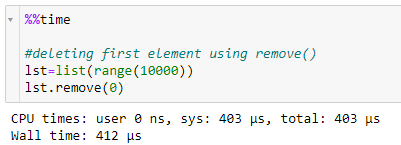Note that` list(range(10000))`  generates a list with numbers `0 to 9999` something like `[0,1,2,3,4,.......9999]`.

Case 2: To delete an element ‘`5000`‘ from the list of 10000 elements using remove().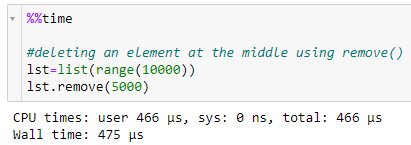Case 3: To delete the last element from a list of 100 elements using remove().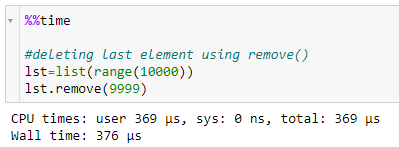## pop()

The `list.pop()` method removes and returns the last element from an existing `list`. The `list.pop(index)` method with the optional argument `index` removes and returns the element at the position `index`.

Syntax: `list.pop(index=-1)`
⦾ where, list is the name of your list.
⦾ index is the index of the element that is to be removed. If the index is not specified, by default, -1 is passed to the method(i.e the last element is removed from the list).

Example 1: Let us say we don’t pass any values to `pop()`, the last element from the list is removed.

```li = [3, 5, 7, 2, 6, 4, 8]
print(li.pop())

# 8```

Note that the deleted value is returned when you call the `pop()` method.

Example 2: Let us say, we pass a value to the `pop()` method, the element located in that index will be deleted.

```li = [3, 5, 7, 2, 6, 4, 8]
print(li.pop(2))

# 7```

• `pop()` acts upon the index of an element.
• `pop() `is a method of the class list.
• `pop()` method returns back the element that is deleted.
• If you specify a value that is not present in the list, Python raises IndexError.
```li = [3, 5, 7, 2, 6, 4, 8]
print(li.pop(8))

# IndexError: pop index out of range```

Complexity Analysis: The computational complexity when removing an `element in index i `from the` list of n elements` using `pop()` method is O(n-i)

Case 1:To delete the first element from a list of 10000 elements using pop().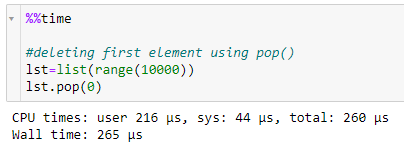Case 2: To delete an element at 4900th index(element 5000, refer to the time complexity example of remove()) from the list of 10000 elements using pop().

Case 3: To delete the last element from a list of 10000 elements using pop().

## del

This is a simple statement in Python that can be used to delete either an element within a class object or a part of the class object or the class object itself. Since a list is a class, any list that we create(eg, `lst=[1,2,3]`) becomes an object of this class list. Hence, del can be used on this object. In simple words, the keyword del can be used to delete an item from the list or a part of the list or the entire list.

Syntax: del `target_list`
⦾ where, target_list can either be an element of the list or the part of the list, or an entire list.

Example 1: To delete an element within a list.

```li = [3, 5, 7, 2, 6, 4, 8]
del li
print(li)

# [5, 7, 2, 6, 4, 8]```

Example 2: To delete a part of the list.

```li = [3, 5, 7, 2, 6, 4, 8]
del li[2:]
print(li)

# [3, 5]```

Example 3: To delete an entire list.

```li = [3, 5, 7, 2, 6, 4, 8]
del li
print(li)

# NameError: name 'li' is not defined```

Note that the list lst got deleted and when we called that there was an error.

• `del` acts upon the index of an element.
• `del` is a keyword that can be used on any class object like lists, dictionaries, tuples, etc.
• `del` does not return anything.
• If you specify a wrong list name or the list name that has already been deleted, it raises a `NameError`.

If you specify an element with the wrong index, Python raises an `IndexError`.

```li = [3, 5, 7, 2, 6, 4, 8]
del li
print(li)

# IndexError: list assignment index out of range```

The computational complexity when removing an element in index i from the list of n elements using del is O(n-i).

Case 1: To delete the first element from a list of 10000 elements using del.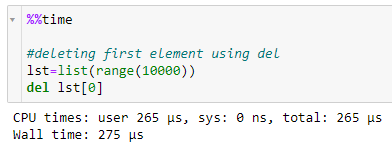Case 2: To delete an element at 4900th index(element 5000, refer to the time complexity example of `remove()`) from the list of 10000 elements using del.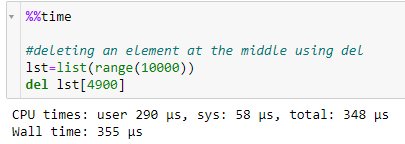Case 3: To delete the last element from a list of 10000 elements using del.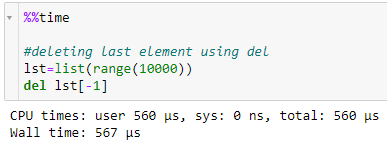## Summary

We hope this article has given some insights on how the three methods work and when each one of them is to be used. Here is a quick summary :

• When you want to delete an element of the list based on it’s value, use `remove()`
• When you want to delete an entire list or a part of the list, use `del`.
• When you want to delete an element of the list based on its index.
• use `pop()` to delete a value that falls somewhere at the end of the list.
• use `pop()` or del for other cases.

For more tutorials and discussions, please subscribe and stay tuned. Thank you for reading. Happy learning!?